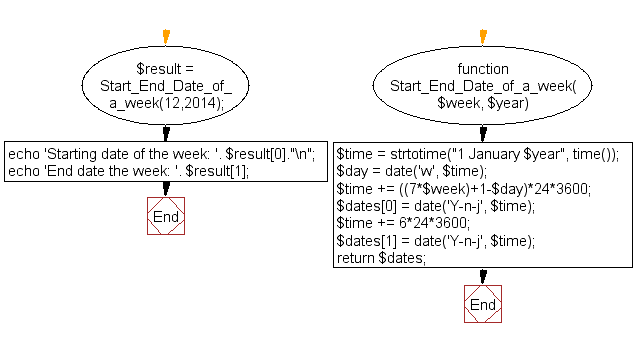﻿ PHP Date Exercise: Start and end date of a week(by week number) of a particular year - w3resource# PHP Date Exercises : Start and end date of a week (by week number) of a particular year

## PHP date: Exercise-17 with Solution

Write a PHP function to get start and end date of a week (by week number) of a particular year.

Sample week and year : 12, 2014

Sample Solution:

PHP Code:

``````<?php
function Start_End_Date_of_a_week(\$week, \$year)
{
\$time = strtotime("1 January \$year", time());
\$day = date('w', \$time);
\$time += ((7*\$week)+1-\$day)*24*3600;
\$dates = date('Y-n-j', \$time);
\$time += 6*24*3600;
\$dates = date('Y-n-j', \$time);
return \$dates;
}

\$result = Start_End_Date_of_a_week(12,2014);
echo 'Starting date of the week: '. \$result."\n";
echo 'End date the week: '. \$result;
?>
```
```

Sample Output:

```Starting date of the week: 2014-3-24
End date the week: 2014-3-30
```

Flowchart :PHP Code Editor:

Have another way to solve this solution? Contribute your code (and comments) through Disqus.

What is the difficulty level of this exercise?

﻿

## PHP: Tips of the Day

SQL injection that gets around mysql_real_escape_string()

Consider the following query:

```\$iId = mysql_real_escape_string("1 OR 1=1");
\$sSql = "SELECT * FROM table WHERE id = \$iId";
```

mysql_real_escape_string() will not protect you against this. The fact that you use single quotes (' ') around your variables inside your query is what protects you against this. The following is also an option:

```\$iId = (int)"1 OR 1=1";
\$sSql = "SELECT * FROM table WHERE id = \$iId";
```

Ref : https://bit.ly/32q3bJ7